﻿ 多跨索道的可移动中间支架设计森林与环境学报2016, Vol. 36Issue (01): 104-110PDF
http://dx.doi.org/10.13324/j.cnki.jfcf.2016.01.017
0

#### 文章信息

ZHOU Chengjun, WU Zhilong, ZHOU Xinnian, FENG Huirong, ZHENG Shifei, LIU Fuwan

Design of movable intermediate supportfor multi-span cableway

Journal of Forest and Environment, 2016, 36(01): 104-110.
http://dx.doi.org/10.13324/j.cnki.jfcf.2016.01.017

### 文章历史

Design of movable intermediate supportfor multi-span cableway
ZHOU Chengjun, WU Zhilong, ZHOU Xinnian, FENG Huirong, ZHENG Shifei, LIU Fuwan
College of Transportation and Civil Engineering, Fujian Agriculture and Forestry University, Fuzhou, Fujian 350002, China
Abstract: Because of the immovable intermediate support,the development of traditional cableway was restricted.In order to achieve the flexibility and economy of multi-span cableway,it was urgently needed to develop a cableway with variable span distances,and the key technology was the movable intermediate support of the multi-span cableway. The main idea of intermediate support lied in how to achieve the movement of the intermediate support in the bracket. Taking a multi-span cableway within a pre-construction as a basis,the feasible movable intermediate support scheme was selected,and the working principle of the selected scheme was elaborated. The design creatively combined the universal wheel and intermediate support bracket,so as to realize the movement of the intermediate support bracket. When the intermediate support did not need to move, the device of jack-up performed to support the bracket,and there was no force acting to the universal wheel.Meanwhile the structure of the main components of movable intermediate support was designed and calculated. At last,an 1:25 physical model was built,which could demonstrate the moving principle of multi-span cableway intermediate support,and the feasibility of the movable intermediate support was verified based on the model,the result showed that the intermediate support satisfied the requirements.
Key words: multi-span cableway    movable intermediate support    design    physical model

1 可移动中间支架的设计思路

2 可移动中间支架的工作原理

3 可移动中间支架的适用范围

4 可移动中间支架的设计计算

 钢索类型Type of cable 单位长度重力Gravity per unit length/(N·m -1) 钢索许可破断拉力Cable permit breaking force/N 横截面面积Cross sectional area/mm 2 钢索弹性模量Cable elastic modulus/MPa 承载索Skyline 24 6×19 NFC 1570 B ZZ 20.20 278 000 229.09 1.0×10 5 起重索Liftline 12 6×19 NFC 1570 B ZS 5.05 69 400 57.27 1.0×10 5 牵引索Haulline 10 6×19 NFC 1570 B ZS 3.51 48 200 37.83 1.0×10 5

 设计参数Design parameters 技术要求Technical requirements 设计吊重Design weight P 1 20 000 N 跑车Carriage K 1跑车:跑车轮数4个，鞍座处安装有托索器K 1 carriage: 4 wheels of a carriage with a cable supporter at saddle 绞盘机Winch machine JSD3-2绞盘机，起重牵引速度 V=1.0 m·s -1Winch machine JSD3-2, lifting speed: V=1.0 m·s -1 无荷中挠系数No charge in torsion coefficients S 0M=0.03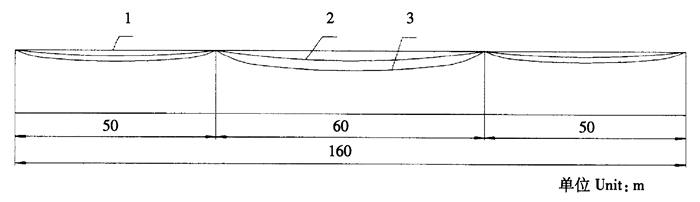注：1.各跨索道弦线；2.索道各跨无荷线形；3.索道各跨有荷线形。Notes:1.each cross line; 2.the cross line of the cable without loading; 3.the cross line of the loading cable. 图 1 索道侧型图 Figure 1 A side of the cableway

4.1 支架受力计算

 $T' = {T_{\max }} + {T_{1\max }} = 132546 + 5388 = 137934{\text{N}}$ (1)

4.2 各跨弯挠角计算

 tanθ1=tanα1-4S01=tan0°-4×0.025=-0.1 (2)
 θ1=-5.71° tanθ2=tanα2+4S02=tan0°+4×0.03=0.12 θ2=6.84° (3)

4.3 T′的水平分力H′和竖直分力V

 H′=T′cosθ2-T′cosθ1=137 934×(cos6.84°-cos5.71°)=298 N (4)
 V′=T′sinθ2+T′sinθ1=137 934×[sin6.84°+sin(-5.71°)]=2 704 N (5)

4.4 支架受到的水平分力H″和竖直分力V

 H″=298 N (6)
 V″=V′+P=2 704+25 417=28 121 N (7)

5 可移动中间支架的强度校核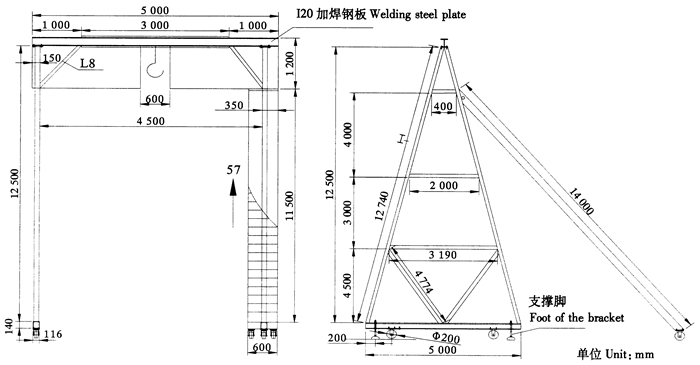图 2 中间支架示意图 Figure 2 Sketch of movable intermediate support
5.1 横梁计算

 Qmax=12F=0.5×63 542=31 771 N (8)

 Mmax=14FL=14×63 542×4.5=71 485 N·m (9)

5.2 内力计算

I20型钢：截面积A=3.55 78×103 mm2，弯曲截面系数Wz=2.37×105 mm3，加焊钢板后：Wz=4.57×105 mm3，Q235钢的许可抗压强度[σ]=170 MPa、许可剪应力[τ]=85 MPa[6, 7]

 $\sigma = \frac{{{M_{\max }}}}{W} = \frac{{71485}}{{457 \times {{10}^{ - 6}}}} = 156.4{\text{MPa < }}\left[\sigma \right] = 170{\text{MPa}}$ (10)
 $\begin{gathered} \hfill \\ \tau = \frac{{3Q}}{{2A}} = \frac{{3 \times 31771}}{{2 \times 35.578 \times {{10}^{ - 4}}}} = 13.39{\text{MPa < }}\tau = 85{\text{MPa}} \hfill \\ \end{gathered}$ (11)

5.3 支腿桁架计算

 $\sigma = \frac{{{F_N}}}{A} = \frac{{86000}}{{13.345 \times {{10}^{ - 4}}}} = 60{\text{MPa < }}\left[\sigma \right] = 170{\text{MPa}}$ (12)

Q235钢长细比$\lambda = \frac{{{L_0}}}{i} = \frac{{5119}}{{98.1}} = 52.18$，故杆件轴心受压构件稳定系数φ=0.865。

 ${\sigma _{\max }} = \frac{{{F_{\max }}}}{{\varphi A}} = \frac{{8600}}{{0.865 \times 14.345 \times {{10}^{ - 4}}}} = 69.3 \leqslant \left[\sigma \right]$ (13)

5.4 支架静止时的受力计算

 G=m·g=(186.7+1 618)×9.81 N=17 704 N (14)

 f=μ·G=0.6×11 704 N=7 022.4 N>298 N (15)

6 万向轮选择及支撑脚计算

6.1 轮压计算

 $最大轮压(满载)P满max = \frac{{15872.6 + 1831.5}}{4} + \frac{{1442.5 + 25417}}{2} = 17855.8N$ (16)
 $P最小轮压(空载)P空min = \frac{{15872.6 + 1831.5}}{4} = 4426N$ (17)

17 855.8 N < 20 000 N，故该万向轮的选择符合要求。

6.2 支撑脚设计计算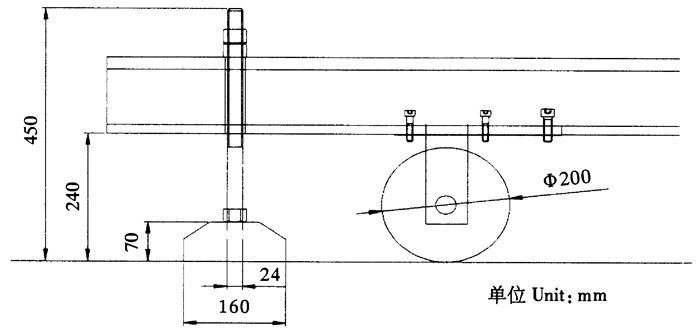图 3 支撑脚和万向轮局部图 Figure 3 Local view of the support foot and universal wheels

 $\sigma = \frac{{3{F_{\max }}h}}{{\pi {b^2}z}} = \frac{{3 \times 1819.2 \times 12}}{{\pi \times {{11}^2} \times 10}} = 17.2{\text{MPa < }}\left[\sigma \right]$ (18)

7 鞍座移动支架的实物模型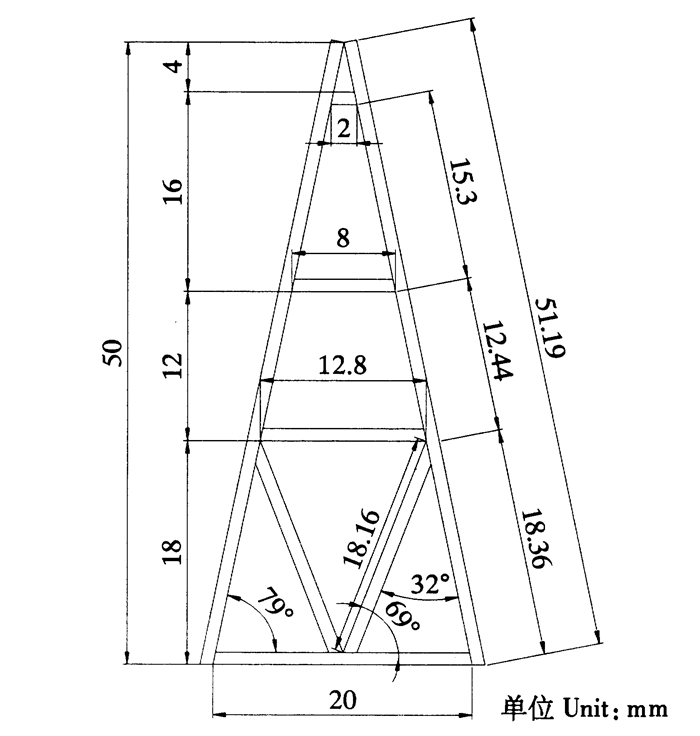图 4 中间支架钢构模型图 Figure 4 Steel model of movable intermediate support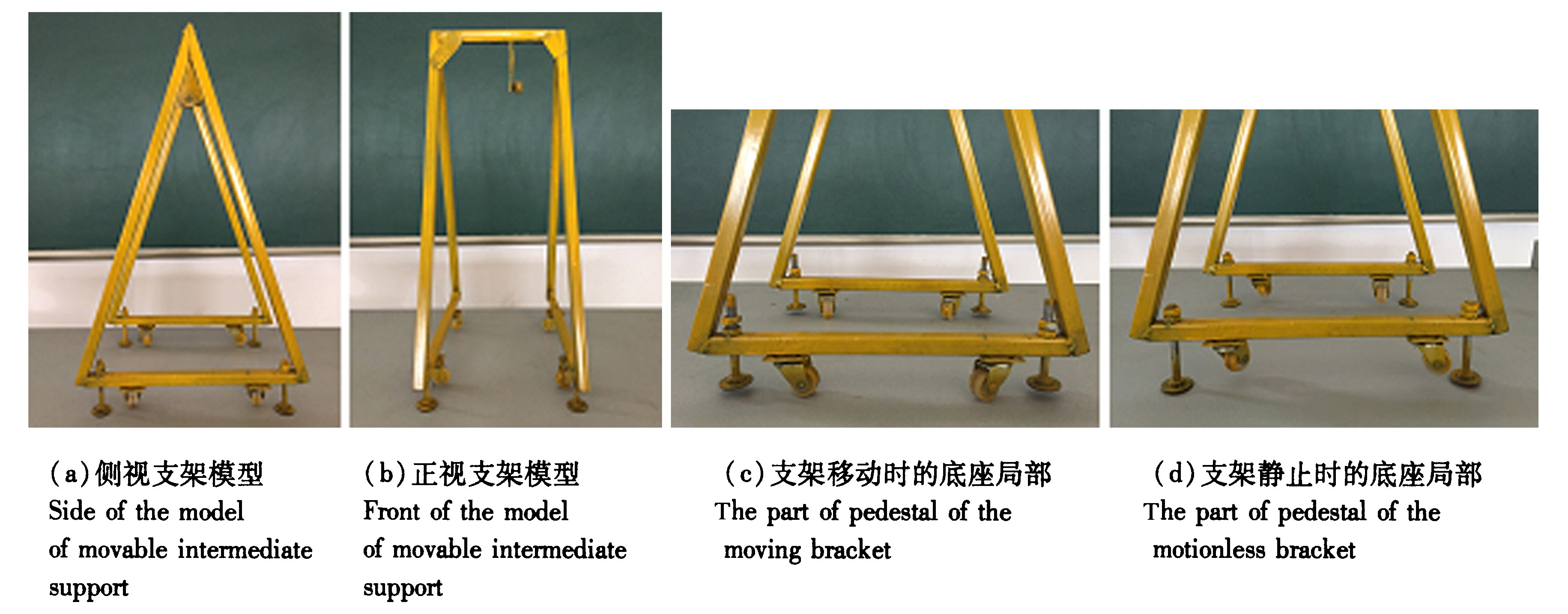图 5 中间支架钢构模型工作示意图 Figure 5 Working sketch of steel model of movable intermediate support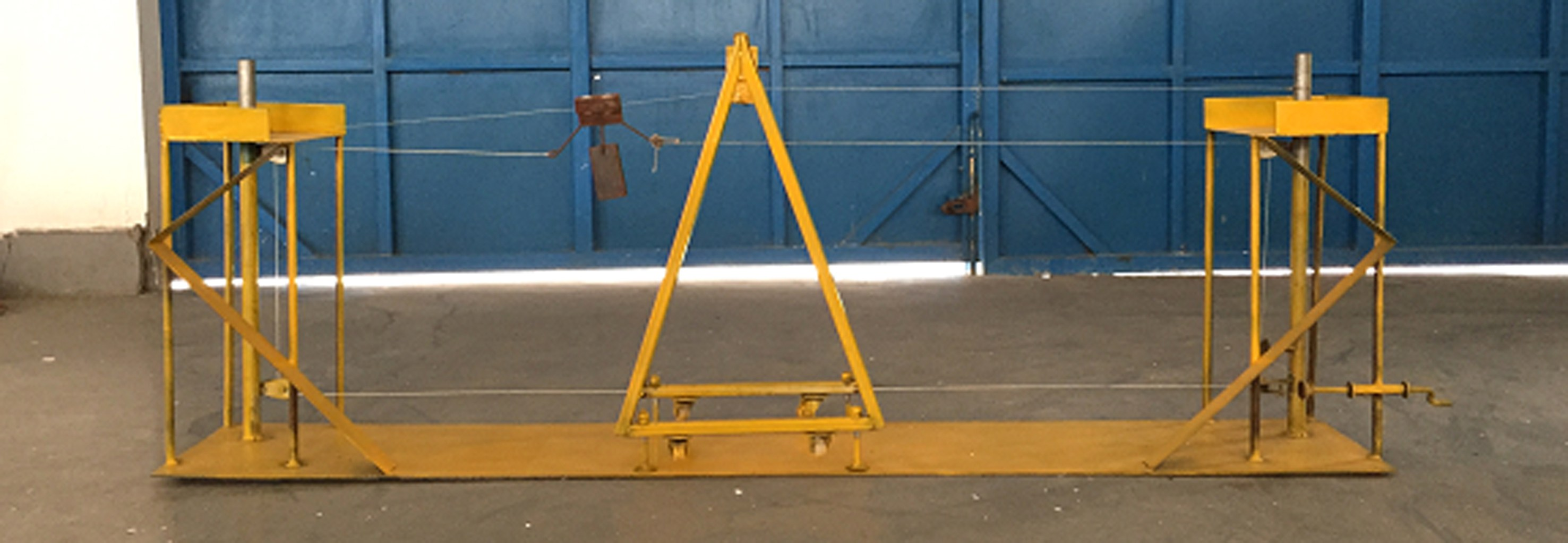图 6 整体索道与鞍座组合模型图 Figure 6 Cableway and movable intermediate support model

8 结论与讨论

  王小桃.多跨单荷重架空索道悬链线理论研究[D].福州:福建农林大学,2012.  周新年,赖阿红,周成军,等.山地森林生态采运研究进展[J].森林与环境学报,2015,35(2):185-192.  金松安.架空索道塔架现代设计方法应用研究[D].重庆:重庆大学,2006.  周新年,巫志龙,周成军,等.工程索道创新训练平台的规划建设[J].森林工程,2014,30(4):192-196.  周新年.工程索道与悬索桥[M].北京:人民交通出版社,2013:169-218.  严自勉.缆索起重机[M].北京:中国电力出版社,2010:41-52.  干光瑜.建筑力学(第2分册):材料力学[M].北京:高等教育出版社,2006:212-220,321.  杨文渊.路桥施工常用数据手册[M].北京:人民交通出版社,2001:111-113.